StatLect

# Exponential distribution - Maximum Likelihood Estimation

In this lecture, we derive the maximum likelihood estimator of the parameter of an exponential distribution. The theory needed to understand this lecture is explained in the lecture entitled Maximum likelihood.## Assumptions

We observe the firstterms of an IID sequenceof random variables having an exponential distribution. A generic term of the sequencehas probability density function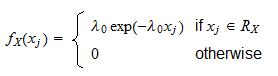whereis the support of the distribution and the rate parameteris the parameter that needs to be estimated.

We assume that the regularity conditions needed for the consistency and asymptotic normality of maximum likelihood estimators are satisfied.

## The likelihood function

The likelihood function isProof

Since the terms of the sequence are independent, the likelihood function is equal to the product of their densities:Because the observed valuescan only belong to the support of the distribution, we can write## The log-likelihood function

The log-likelihood function isProof

This is obtained by taking the natural logarithm of the likelihood function: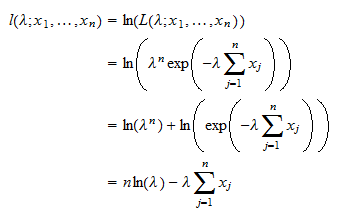## The maximum likelihood estimator

The maximum likelihood estimator ofisProof

The estimator is obtained as a solution of the maximization problemThe first order condition for a maximum isThe derivative of the log-likelihood is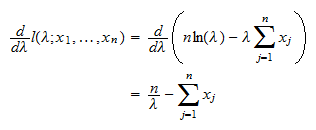By setting it equal to zero, we obtainNote that the division byis legitimate because exponentially distributed random variables can take on only positive values (and strictly so with probability 1).

Therefore, the estimatoris just the reciprocal of the sample mean## Asymptotic variance

The estimatoris asymptotically normal with asymptotic mean equal toand asymptotic variance equal to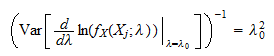Proof

The score is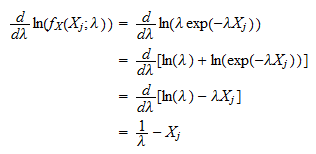The Hessian isBy the information equality, we have thatFinally, the asymptotic variance is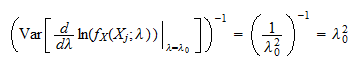This means that the distribution of the maximum likelihood estimatorcan be approximated by a normal distribution with meanand variance.

The book

Most of the learning materials found on this website are now available in a traditional textbook format.

Glossary entries
Share